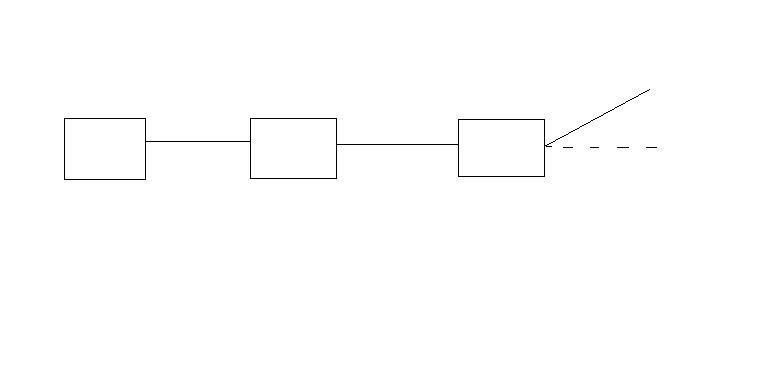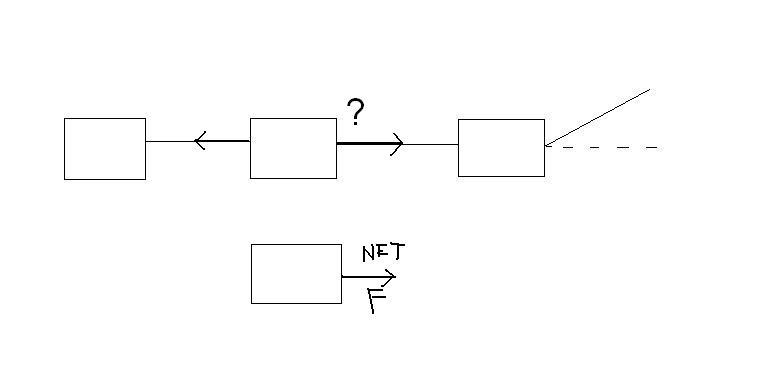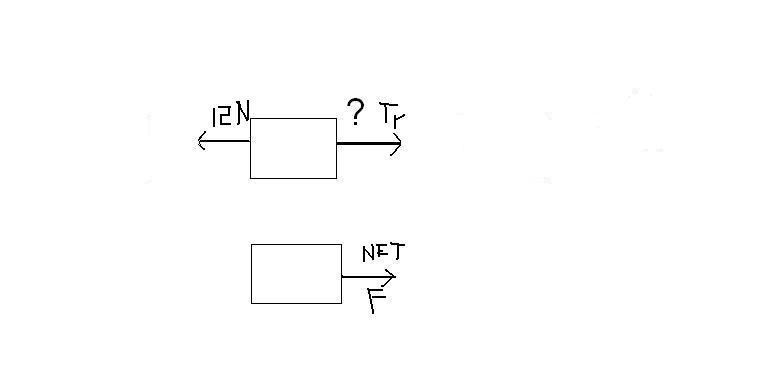# Tension in the cord problem

## Homework Statement

A worker pulls 3 carts connected with 2 horizontal cords. The mass of the last cart is 16.1kg, the middle cart's is 13.2kg and the first cart's is 15.0kg. Friction is negligible. A third cord which pulls on the first cart is at an angle of 21.0 degrees above the horizontal, has a tension of magnitude 35.3N. Determine the magnitude of
a) the acceleration of the carts
b)the tension in the last cord
c) the tension in the middle cord.

sigma F= ma

## The Attempt at a Solution

a) I tried using Newton's 2nd law equation to find acceleration by using the tension in the cord as the force, but I don't get the write answer.
35.3N = (16.1kg + 13.2kg + 15.0kg)a
0.797m/s squared
I then tried finding the horizontal force pulling on the carts instead of the force of the tension of the rope which was at an angle of 21 degrees above the horizontal.
cos 21 = Fb/35.3N
Fb = 32.955 N
32.955N = (44.3kg)a
0.744m/s squared= a
I still get it wrong.
The correct answer is 0.744 m/s squared.
b) and c) I have no idea what I'm doing there.

Last edited:

## Homework Statement

A worker pulls 3 carts connected with 2 horizontal cords. The mass of the last cart is 16.1kg, the middle cart's is 13.2kg and the first cart's is 15.0kg. Friction is negligible. A third cord which pulls on the first cart is at an angle of 21.0 degrees above the horizontal, has a tension of magnitude 35.3N. Determine the magnitude of
a) the acceleration of the carts
b)the tension in the last cord
c) the tension in the middle cord.

sigma F= ma

## The Attempt at a Solution

a) I tried using Newton's 2nd law equation to find acceleration by using the tension in the cord as the force, but I don't get the write answer.
35.3N = (16.1kg + 13.2kg + 15.0kg)a
0.797m/s squared
I then tried finding the horizontal force pulling on the carts instead of the force of the tension of the rope which was at an angle of 21 degrees above the horizontal.
cos 21 = Fb/35.3N
Fb = 32.955 N
32.955N = (44.3kg)a
0.744m/s squared= a
I still get it wrong.
The correct answer is 0.744 m/s squared.
b) and c) I have no idea what I'm doing there.

Can you draw free body diagrams of the forces that act on each cart?

a) does not actually require this, but it is helpful for the others to help you see how to solve them.

You got a) correct, what do you not understand on a?

Last edited:

Do i just write it out here? Well,
35.3N
/
| Fn | Fn | Fn /
| | | / 21 degrees
16.1 kg -------- 13.2kg --------- 16.1kg ______________________
| | | 32.955N
|Fg |Fg |Fg

Well... that didn't end up too well...
But it's just the horizontal force we're looking at isn't it? Do the normal and gravity forces affect the acceleration?

Well... that didn't end up too well...
But it's just the horizontal force we're looking at isn't it? Do the normal and gravity forces affect the acceleration?

Not in this case. Its all the horizontal forces, correct. Lets start here: Why do you think you got a) wrong, it looks correct?

Oh... wait a minute a) is right >_> I just looked at the wrong answer.
so to find the tension of the last rope do i just do this:? F= 16.1kg(0.744) = 11.97N
and for the middle rope do i just do the same thing, but use the mass of bot the second cart and the last cart?

Oh... wait a minute a) is right >_> I just looked at the wrong answer.
so to find the tension of the last rope do i just do this:? F= 16.1kg(0.744) = 11.97N
and for the middle rope do i just do the same thing, but use the mass of bot the second cart and the last cart?

Yep.
Let me ask you this. How many forces are acting on the last cart in the horizontal?

3? Gravity, normal force and the force applied?

3? Gravity, normal force and the force applied?

Ok. But only one in the horizontal and its Tension, what you are calling the applied force and you reasoned that the last cart accelerates the same as all the other carts so you got it.

So, how many forces and in what direction do these forces act on the second cart, the 13.2 kg cart?

Are there not two ropes attached to the 2nd cart and they "pull" opposite each other, yes?

The tension from the first cord and the tension of the cord connecting the last cart to it?

The tension from the first cord and the tension of the cord connecting the last cart to it?

So yes, the tension from the cord attached to the last cart, and the tension of the cord attached to the 1st cart, right? Are there not 3 cords?

yes.

yes.

So a picture would look like this....If so lets now deal with the 2nd cart and the two ropes attached and the net force on the 2nd cart.

Last edited:

Yeah

Yeah

So what is the net force on the middle cart (2nd cart) since it is accelerating? F = ma

What number do you get in Newtons?

Last edited:

F = (16.1 + 13.2) (0.744) = 11.98N
Is that how you do it?

F = (16.1 + 13.2) (0.744) = 11.98N
Is that how you do it?

Hold on. What is the mass of just the 2nd cart and what is its acceleration? You know both of these, one was given (mass,) and one you already determined, you just thought you got it wrong. ALL the carts must have the same acceleration, yes?

The mass of the 2nd cart is 13.2kg and its accleration is 0.744m/s squared.

The mass of the 2nd cart is 13.2kg and its accleration is 0.744m/s squared.

Yes.
So you know the net force. 13.2 * 0.744

So what leads to that net force. There are two forces acting. You already calculated one of them. These two forces are both tension. Look at the picture. You know the force to the left and you know the net force which is to the right. So the force of tension to the right, which is the answer to the last part of your question must be what?
Take a look at the picture, maybe you can see it better visually.The force on the left is 12.0N the force on the right is 32.955N
so the net force is 32.955N - 12N = 23.13N?

The force on the left is 12.0N the force on the right is 32.955N
so the net force is 32.955N - 12N = 23.13N?

The force on the left is correct you got that in part b)

The net force is just F = ma or 13.2 x .744

So the force on the right the one with the ? mark must beat out the force on the left, the 12N by the net force, yes? So with math symbols T(right) - T (left) = overall force or net force... which is to the right.

Does this make sense? Try this and what do you get?

This bit of picture from the other picture...It could also be written T (right) = T(left) + net force

So T(right) - 12N = 9.8208N
T(right) = 21.8

Because the net force i just T(right) = T(left) right?

So T(right) - 12N = 9.8208N
T(right) = 21.8

Because the net force i just T(right) = T(left) right?

Do you mean the net force = T (right) - T (left) ?

T (right) has got to be bigger than T (left) if the 2nd cart accelerates to the right, thus the net force is to the right.